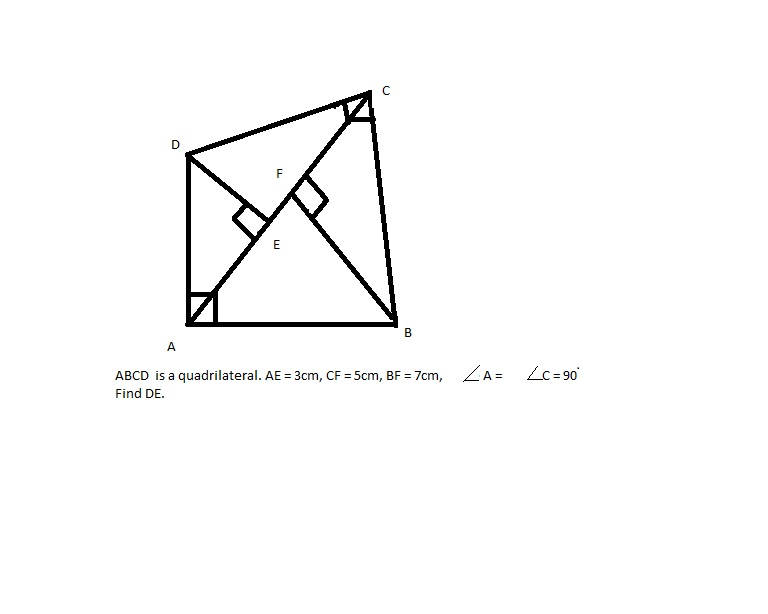1800-212-7858 (Toll Free)
9:00am - 8:00pm IST all days
8104911739

or

Thanks, You will receive a call shortly.
Customer Support

You are very important to us

022-62211530

Mon to Sat - 11 AM to 8 PM

Ask Congruence Criteria Sss And Rhs question free ×

Queries asked on Sunday and after 7 pm from Monday to Saturday will be answered after 12 pm the next working day.

• CBSE×
• Class 9×
• Mathematics×
• Triangles×
• Congruence Criteria Sss And Rhs×
Home /Doubts and Solutions/CBSE/Class 9/Mathematics/Triangles/Congruence Criteria Sss And Rhs

Congruence Criteria Sss And Rhs Free Doubts and Solutions

How to proove RHS congruence

Asked by Nimmi 26th January 2019, 5:10 PM

×

If the altitude drawn  from the vertices of triangle ABC  to opposite sides are equal, prove that the triangle is equilateral .

Asked by nisha_vini29 27th February 2018, 11:37 PM

×

State the congruence criterion for the triangles to be congruent in figure (i) and (ii) Write the correct correspondence for each.

Asked by Topperlearning User 10th August 2017, 12:46 PM

×

In an isosceles triangle, prove that the altitude from the vertex divides the triangle into two congruent triangles..

Asked by Topperlearning User 10th August 2017, 12:46 PM

×

ABC is an isosceles triangle with AB = AC. Bisectors of B and C intersect at O. Prove that BO = CO and AO bisects BAC.

Asked by Topperlearning User 10th August 2017, 12:46 PM

×

ABCD is a parallelogram, if the two diagonals are equal, find the measure of ABC.

Asked by Topperlearning User 10th August 2017, 12:45 PM

×

ABCD is a square, X and Y are the points on the sides AB and DC respectively such that BY = CX. Prove that CY = BX and CBY = BCX.

Asked by Topperlearning User 10th August 2017, 12:44 PM

×

If two isosceles triangles have a common base, then the line joining their vertices bisects the base at right angles.

Asked by Topperlearning User 10th August 2017, 12:44 PM

×

In quadrilateral PQRS the diagonals intersect at O than prove that PQ + QR + RS + PS > PR + QS.

Asked by Topperlearning User 10th August 2017, 12:41 PM

×

CBSE - IX - Mathematics - Triangles

Asked by Topperlearning User 10th August 2017, 12:40 PM

×

CBSE - IX - Mathematics - Triangles

Asked by Topperlearning User 10th August 2017, 12:39 PM

×

ABCD is a parallelogram whose diagonals is equal in length and intersects at right angles. Prove that, ABCD is a square.

Asked by Topperlearning User 10th August 2017, 12:38 PM

×

ABCD is a quadrilateral in which AB = AD, BC = DC. AC and BD intersect at E. Prove that, AC bisects each of the angles A and C.

Asked by Topperlearning User 10th August 2017, 12:38 PM

×

A point O is taken inside an equilateral four sided figure ABCD such that its distances from the angular points D and B are equal. Show that AO and OC are in one and the same straight line.

Asked by Topperlearning User 10th August 2017, 12:36 PM

×

AD is the median of ABC. If BL and CM are drawn perpendiculars on AD produced, prove that BL= CM.

Asked by Topperlearning User 10th August 2017, 12:36 PM

×

In the given figure, BAAC and DEEF such that BA = DE and BF = DC. Prove that AC = EF.

Asked by Topperlearning User 10th August 2017, 12:35 PM

×

CBSE - IX - Mathematics - Triangles

Asked by Topperlearning User 10th August 2017, 12:34 PM

×

show that in a right angled triangle, the hypotenuse is the longest side.

Asked by mahendra_soniya 17th August 2015, 6:15 PM

×

ABC is a triangle in which angle b = 2 angle c. D is a point on side bc such that add bisectes angle bac. and ab = cd. Find the measure of angle bac Ans:Vatsal Choudhary In this solution provided by meritnation please tell me how angle acd = angle dac and how ab = cd

Asked by vatsalchoudhary41 25th December 2014, 3:45 PM

×Asked by araima2001 4th July 2014, 5:06 PM

×

In fig., A is a point equidistant from two lines l1 and l2 intersecting at a point P. Show that AP bisects the angle between l1 and l2.

Asked by Topperlearning User 4th June 2014, 1:23 PM

×

Two sides AB and BC and median AM of ABC are respectively equal to the sides PQ and QR and median PN of PQR. Show that ABM PQN.

Asked by Topperlearning User 4th June 2014, 1:23 PM

×

In the following figure, AD is a median of and BL and CM are perpendiculars drawn from B and C respectively on AD and AD produced respectively. Prove That BL=CM.

Asked by Topperlearning User 4th June 2014, 1:23 PM

×

In figure, D is the mid-point of base BC, DE and DF are perpendiculars to AB and AC respectively such that DE = DF. Prove that B = C.

Asked by Topperlearning User 4th June 2014, 1:23 PM

×

In figure, AB = AC, D is the point in the interior of ABC such that DBC = DCB. Prove that AD bisects BAC of ABC.

Asked by Topperlearning User 6th December 2013, 2:12 AM